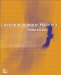# Appendix A: Mathematical Review

Much of the research literature available to the network designer may often appear impenetrable, since the language used to express ideas involves advanced mathematical notation. Although this book is not heavily numerical, it is assumed that the reader has a basic grasp of mathematics, as the use of mathematical conventions in some areas of network design is unavoidable. In this appendix we briefly review a selection of the more common techniques applicable to data communications design theory.

## A.1 Operators

 + Add - Subtract = Equals ≅ or ≈ Approximately equal to > Greater than ≥ Greater or equal to √ The square root of × Multiply ÷ Divide ≠ Not equal to < Less than ≤ Less than or equal to ^ Raised to the power of → Tends to or approachesActionScripting in Flash MX
ISBN: N/A
EAN: 2147483647
Year: 2001
Pages: 117